Courses
Courses for Kids
Free study material
Free LIVE classes
More

# JEE - Limit, Continuity and DifferentiabilityLIVE
Join Vedantu’s FREE Mastercalss

## What is Limit, Continuity and Differentiability?

When we talk about calculus, we frequently notice that limits and continuity have a distinct and essential position due to their extremely different and important notions. The term limit, on the one hand, refers to the number that a function approaches, whereas the term function can be continuous or discontinuous. Positive discontinuity, Jump discontinuity, and Infinite discontinuity are the three types of discontinuity that fit into this category. We'll go through this topic in-depth with the help of solved examples in this part.

## JEE Main Maths Chapter-wise Solutions 2023-24

 JEE Main Maths Chapter-wise Solutions 1 Sets, Relations and Functions 9 Integral Calculus 2 Complex Numbers and Quadratic Equations 10 Differential Equations 3 Matrices and Determinants 11 Co-ordinate Geometry 4 Permutations and Combinations 12 Three Dimensional Geometry 5 Mathematical Induction 13 Vector Algebra 6 Binomial Theorem 14 Statistics and Probability 7 Sequence and Series 15 Trigonometry 8 Limit, Continuity and Differentiability 16 Mathematical Reasoning

### Important Topics of Limit, Continuity and Differentiability

• Limits and Derivatives

• Evaluating Limit

• Algebra of limits

• L’Hospital Rule

• Function

• Continuity and Differentiability

• Continuity and Differentiability Properties and Formula

• Limits Properties and Formula

• Intermediate Value Theorem

• Application of Derivatives

• Maxima and Minima

• Rolle’s Theorem and Lagrange’s Mean Value Theorem

### What are Limits?

The value obtained by a function f(x) at a point x=a is the value obtained by the function at a point very close to x=a.

Consider the limit of a real-valued function ‘f’ and a real number ‘a’, which is generally say that, $\underset{x \to a}{\lim} f(x) = L$ if there is a number $\delta$ for every number $\epsilon$ such that $|f(x)-L| < \epsilon$ whenever $0 < |x-a| < \delta$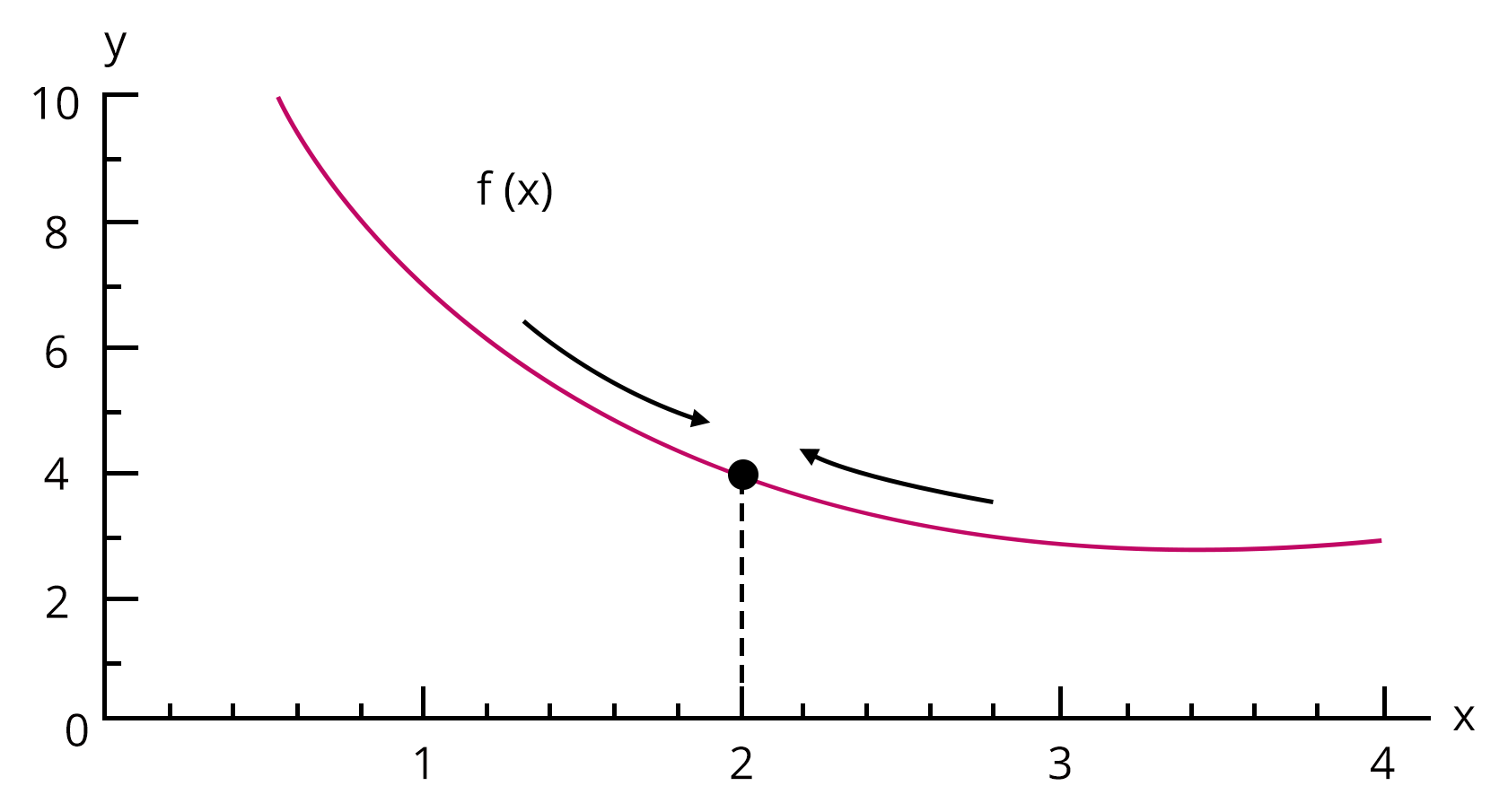### Existence of Limit, Left Hand Limit and Right Hand Limit - Types of Limits

Left Hand Limit - When a limit is described in terms of a number smaller than a, it is referred to as a left hand limit. It's written as $x \to a^{-}$, which is the same as $x=a-h$ with $h > 0$ and $h \to 0$.

Right Hand Limit - When a limit is described in terms of a number greater than a, it is referred to as a right hand limit. It is written as $x \to a^{+}$, which is the same as $x=a+h$ with $h > 0$ and $h \to 0$.

Existence of Limit - A function's limit at $x = a$ exists only if both its left and right hand limits exist, are equal, and have a finite value, i.e., $\underset{x \to a^{-}}{\lim} f(x) = \underset{x \to a^{+}}{\lim} f(x)$

### Limits and Functions

A function may reach one of two limits. One in which the variable approaches its limit by taking values that are greater than the limit, and the other in which the variable approaches its limit by taking values that are smaller. The limit is not stated in this situation, although the right and left-hand limitations do exist.

• When the $\underset{x \to a}{\lim} f(x) = A^{+}$ is used, the values of f near x to the right of ‘a’ are used. The right hand limit of f(x) at ‘a’ is stated to be this value.

• When the $\underset{x \to a}{\lim} f(x) = A^{-}$ is used, the values of f near x to the left of ‘a’ are used. The left hand limit of f(x) at ‘a’ is referred to as this value.

• The function’s limit exists if and only if the left-hand limit is equal to the right-hand limit.

### Important Limits Formula

1. $\underset{x \to 0}{\lim} \dfrac{\sin x}{x}=1$

2. $\underset{x \to 0}{\lim} \cos x=1$

3. $\underset{x \to 0}{\lim} \dfrac{\tan x}{x}=1$

4. $\underset{x \to 0}{\lim} \dfrac{1-\cos x}{x}=0$

5. $\underset{x \to 0}{\lim} \dfrac{\sin x^{\circ}}{x}=\dfrac{\pi}{180}$

6. $\underset{x \to a}{\lim} \dfrac{x^{n}-a^{n}}{x-a}=n a^{n-1}$

7. $\underset{x \to \infty}{\lim}\left(1+\dfrac{k}{x}\right)^{m x}=e^{m k}$

8. $\underset{x \to 0}{\lim}(1+x)^{\dfrac{1}{x}}=e$

9. $\underset{x \to 0}{\lim} \dfrac{\left(a^{x}-1\right)}{x}=\ln a$

10. $\underset{x \to 0}{\lim} \dfrac{e^{x}-1}{x}=1$

11.  $\underset{x \to 0}{\lim} \dfrac{\ln (1+x)}{x}=1$

12.  $\underset{x \to \infty}{\lim} x^{\dfrac{1}{x}}=1$

### L’Hospital Rule

If the supplied limit $\underset{x \to a}{\lim} \dfrac{f(x)}{g(x)}$ is of the form $\dfrac{0}{0}$ or $\dfrac{\infty}{\infty}$, that is, both f(x) and g(x) are 0 or both f(x) and g(x) are infinity, then the limit can be solved using the L'Hospital Rule that is,

$\underset{x \to a}{\lim} \dfrac{f(x)}{g(x)} = \underset{x \to a}{\lim} \dfrac{f^\prime(x)}{g^\prime(x)}$, where $f^\prime(x)$ and $g^\prime(x)$ is obtained by differentiating f(x) and g(x).

### What is Continuity and Differentiability?

The continuity of a function and the differentiability of a function are mutually exclusive. Before it can be shown for its differentiability at x = a, the function y = f(x) must first be proved for its continuity at x = a. Both continuity and differentiability can be demonstrated geometrically and algebraically.

### Continuity of a Function

The graph y = f(x) of a function is said to be continuous over a range if it is a single unbroken curve (the graph can be drawn easily without lifting the pencil at a point).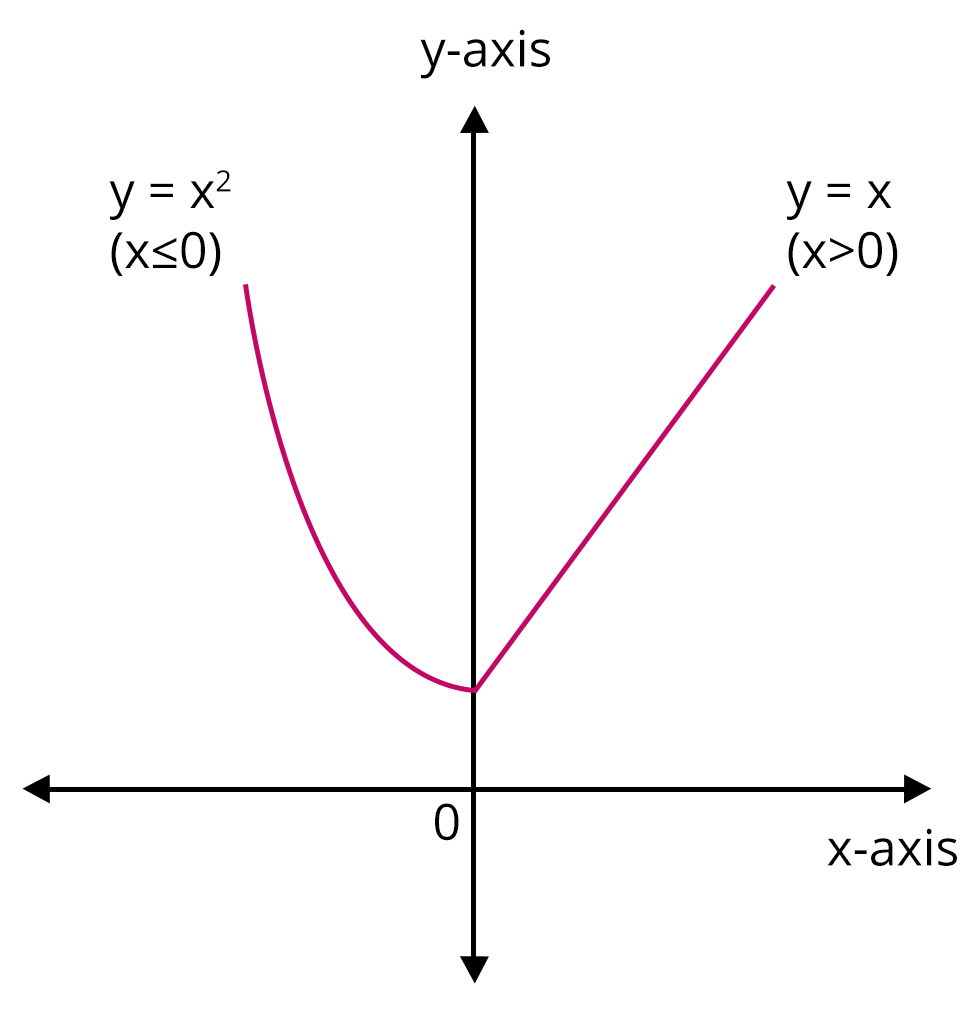Image: Graph of function y = f(x)

Technically we can say that, a continuous real valued function f(x) exists and is equal to $f(x_0)$ at a point $x=x_0$ in the domain if $\underset{x \to x_0}{\lim} f(x)$ exists and is equal to $f(x_0)$.

If a function f(x) is continuous at $x=x_0$, then $\underset{x \to x_0^{+}}{\lim} f(x) = \underset{x \to x_0^{-}}{\lim} f(x) = \underset{x \to x_0}{\lim} f(x)$

Discontinuous functions are those that are not continuous.

### Differentiability of A Function

The derivative of a real valued function f(x) with respect to x if the derivative $f^\prime(a)$ exists at every point in its domain, which is defined as

$\underset{h\to 0}{\lim} \dfrac{f(x+h)-f(x)}{h}$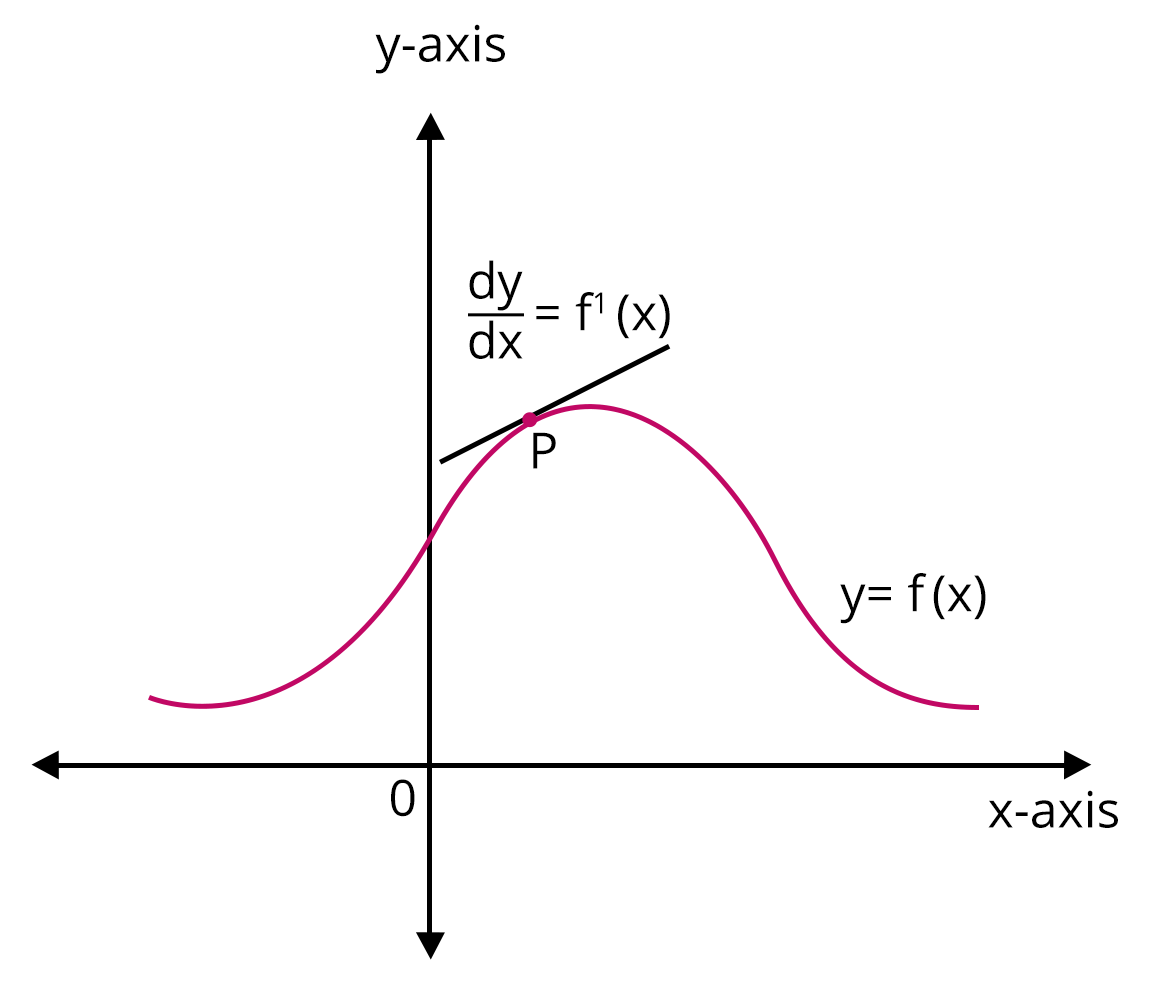Image: Derivative of a function f(x)

If the derivative of a function exists at all points in its domain, the function is said to be differentiable. To test the differentiability of a function at x=c, $\underset{h\to 0}{\lim} \dfrac{f(c+h)-f(c)}{h}$ must exist.

Thus we can say that every differentiable function is continuous

Note: If a function is differentiable at a given point, it is also continuous at that same point. But just because a function is continuous at a point doesn't mean it's also differentiable at that point.

### Important Differentiation of the Functions

Derivative of Composite Function

In Layman’s terms, to differentiate a composite function at any point in its domain, first differentiate the outer part (i.e., the function enclosing some other function) and then multiply it with the inner function’s derivative function. This will provide us with the desired differentiation.

Derivatives of Implicit Functions

When the dependent variable in a function is not explicitly isolated on either side of the equation, the function is referred to as an implicit function.

Derivatives of Inverse Trigonometric Functions

The derivatives of inverse trigonometric functions are known as inverse trigonometric derivatives. The inverses of the six basic trigonometric functions are called inverse trigonometric functions. Inverse trigonometric derivatives are defined only in the domain of inverse trigonometric functions, as follows:

 Inverse Trigonometric Function Domain arcsin x $\left[-1, 1\right]$ arccos x $\left[-1, 1\right]$ arctan x R arccosec x $(-\infty, -1] \cup [1, \infty)$ arcsec x $(-\infty, -1] \cup [1, \infty)$ arccot x R

Derivatives of Exponential Functions

The product of the exponential function ax and the natural log of a, $f^\prime(x) = ax~ln ~a$, is the derivative of the exponential function $f(x) = ax$, $a > 0$. The exponential function's derivative is written as $\dfrac{d(ax)}{dx} = (ax)^\prime = ax~ln~a$. The first principle of differentiation and the formulas of limits can be used to calculate the derivative of an exponential function. When $a > 1$ and when $a < 1$, the graph of the exponential function's derivative changes direction.

Formula for derivative of exponential function is given as,

$f(x) = ax, f^\prime(x) = ax~ln~a$ or $\dfrac{d(ax)}{dx} = ax~ln~a$

$f(x) = ex, f^\prime(x) = ex$ or $\dfrac{d(ex)}{dx} = ex$

Derivatives of Logarithmic Functions

The slope of the tangent to the curve representing the logarithmic function is determined by the logarithmic function's derivation. The following is the formula for the derivative of the common and natural logarithmic functions.

$\dfrac{1}{x}$ is the derivative of $ln x$. For example, $\dfrac{d}{dx} \dfrac{1}{x} = ln x$

$\dfrac{1}{x(x ~log~ a)}$ is the derivative of $log_a x$

Derivatives of Functions in Parametric Forms

There are times when we define a function using the third variable rather than explicitly or implicitly defining it. This is a parametric form, which is when a function y(x) is represented by a third variable called the parameter.

$\dfrac{d^2y}{dx^2} = \dfrac{d}{dx}\left(\dfrac{dy}{dx}\right) = \dfrac{\dfrac{d}{dt} \left(\dfrac{dy}{dx}\right)} {\dfrac{dx}{dt}}$

### Important Formulae of Continuity and Differentiability

1. $f(x)$ is continuous at $x=a$ if $\lim _{x \rightarrow a-} f(x)=\lim _{x \rightarrow a+} f(x)=f(a)$

2. $\dfrac{d}{d x}\left(x^{n}\right)=n x^{n-1}$ i.e $\dfrac{d}{d x}(\mathrm{x})=1$.

3. $\dfrac{d}{d x}(\sin x)=\cos x$.

4. $\dfrac{d}{d x}(\cos x)=-\sin x$.

5. $\cdot \dfrac{d}{d x}(\tan x)=\sec ^{2} x .$

6. $\dfrac{d}{d x}(\cot x)=-\operatorname{cosec}^{2} x$.

7. $\dfrac{d}{d x}(\sec x)=\sec x \tan x$.

8. $\dfrac{d}{d x}(\operatorname{cosec} x)=-\operatorname{cosec} x \cot x$.

9. $\dfrac{d}{d x}\left(\sin ^{-1} x\right)=\dfrac{1}{\sqrt{1-x^{2}}}$

10. $\dfrac{d}{d x}\left(\cos ^{-1} x\right)=-\dfrac{1}{\sqrt{1-x^{2}}}$

11. $\dfrac{d}{d x}\left(\tan ^{-1} x\right)=\dfrac{1}{1+x^{2}}$

12. $\dfrac{d}{d x}\left(\cot ^{-1} x\right)=-\dfrac{1}{1+x^{2}}$.

13. $\dfrac{d}{d x}\left(\sec ^{-1} x\right)=\dfrac{1}{x \sqrt{x^{2}-1}}$

14. $\dfrac{d}{d x}\left(\operatorname{cosec}^{-1} x\right)=-\dfrac{1}{x \sqrt{x^{2}-1}}$

### Theorems on Continuity and Differentiability

Theorem 1: If two functions f(x) and g(x) are continuous at a real valued function and continuous at a point x = c, we have:

At the point $c = c$, $f(x) + g(x)$ is continuous.

At $x = c$, $f(x) - g(x)$ is continuous.

Also at $x = c$, $g(x) \cdot g(x)$ is continuous.

At $x = c$, $\dfrac{f(x)}{g(x)}$ is continuous if $g(c) \neq 0$.

Theorem 2: The composite function fog(x) is defined at x = c for two real-valued functions f(x) and g(x). If the function f(x) is continuous at g(c) and g(x) is continuous at x = c, then fog(x) is continuous at x = c.

Theorem 3: If a function f(x) is differentiable at x = c, it is also continuous at that point. Every differentiable function is continuous, in other words.

Theorem 4: Chain Rule - $f = vou$ for a real-valued function f(x) is a composite of two functions u and v. Also, if $t = u(x)$ and both $\dfrac{dt}{dx}$ and $\dfrac{dv}{dt}$ exist, then $\dfrac{df}{dx} = \dfrac{dv}{dt}\cdot dt \cdot dx$ is obtained.

Theorem 5: The derivative of $e^x$ with respect to $x$ is $e^x$. And the derivative of $log x$ with respect to $x$ is $\dfrac{1}{x}$.

Theorem 6: Rolle's Theorem - If a function f(x) is continuous across the interval $\left[a, b\right]$ and differentiable across the interval (a, b), such that f(a) = f(b), and a, b are some real numbers. Then there exists a point c in the interval $\left[a, b\right]$ such that $f^\prime(c) = 0$.

Theorem 7: Mean Value Theorem - If a function f(x) is continuous across the interval $\left[a, b\right]$ and differentiable across the interval (a, b), then there exists a point c in the interval $\left[a, b\right]$ such that $f^\prime(c) = \dfrac{f(b) - f(a)}{b - a}$.

### Applications of Derivatives:

1. Rate of Change Formula

The rate of change function represents the rate at which one quantity changes in relation to another. Simply put, the rate of change is the difference between the amount of change in one item and the corresponding amount of change in another.

i.e., $\dfrac{\Delta y}{\Delta x} = \dfrac{y_2 - y_1}{x_2 - x_1}$

For a linear function

$\dfrac{\Delta y}{\Delta x} = \dfrac{f(b) - f(a)}{b - a}$

Note:

The rate of change of quantity, $\dfrac{dy}{dx}$, is represented by a positive sign when it increases.

The rate of change of quantity, $\dfrac{dy}{dx}$, is represented by a negative sign when it decreases.

2. Increasing and Decreasing Functions

If the value of y increases as the value of x increases, then the function $y = F(x)$ is known as an increasing function, and if the value of y decreases as the value of x increases, then the function $y = F(x)$ is known as a decreasing function.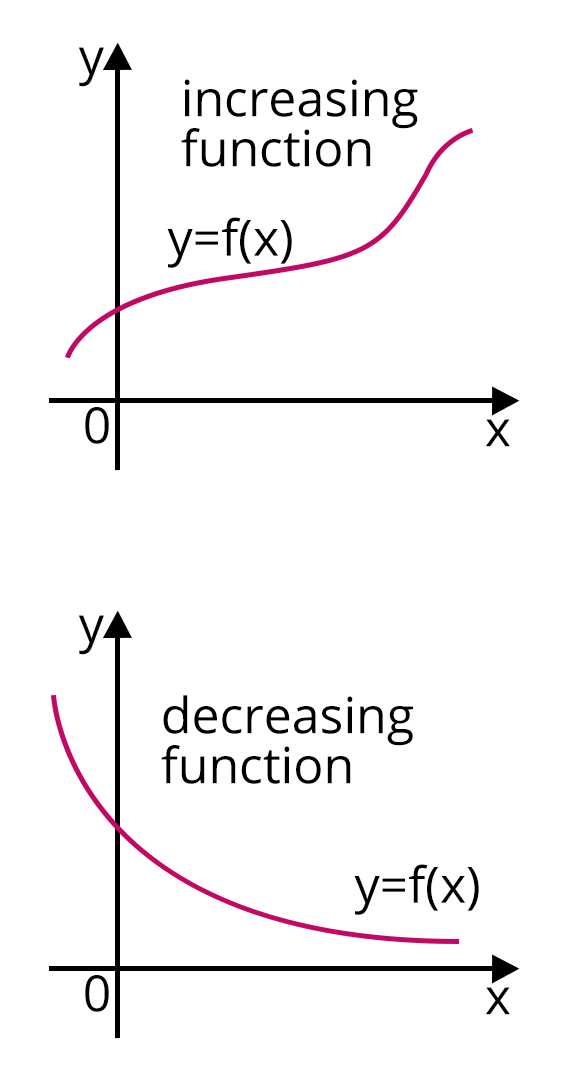Image: Increasing and Decreasing Function

3. Maxima and Minima of Functions

The extrema of a function are its maximum and minimum values. The maximum and minimum values of a function within a given set of ranges are known as maxima and minima. The maximum value of the function under the entire range is known as the absolute maxima, and the minimum value is known as the absolute minima.

In the diagram below, we get maximum values of the function at x = a and x = 0, and minimum values of the function at x = b and x = c. The maxima are all the peaks, and the minima are all the valleys.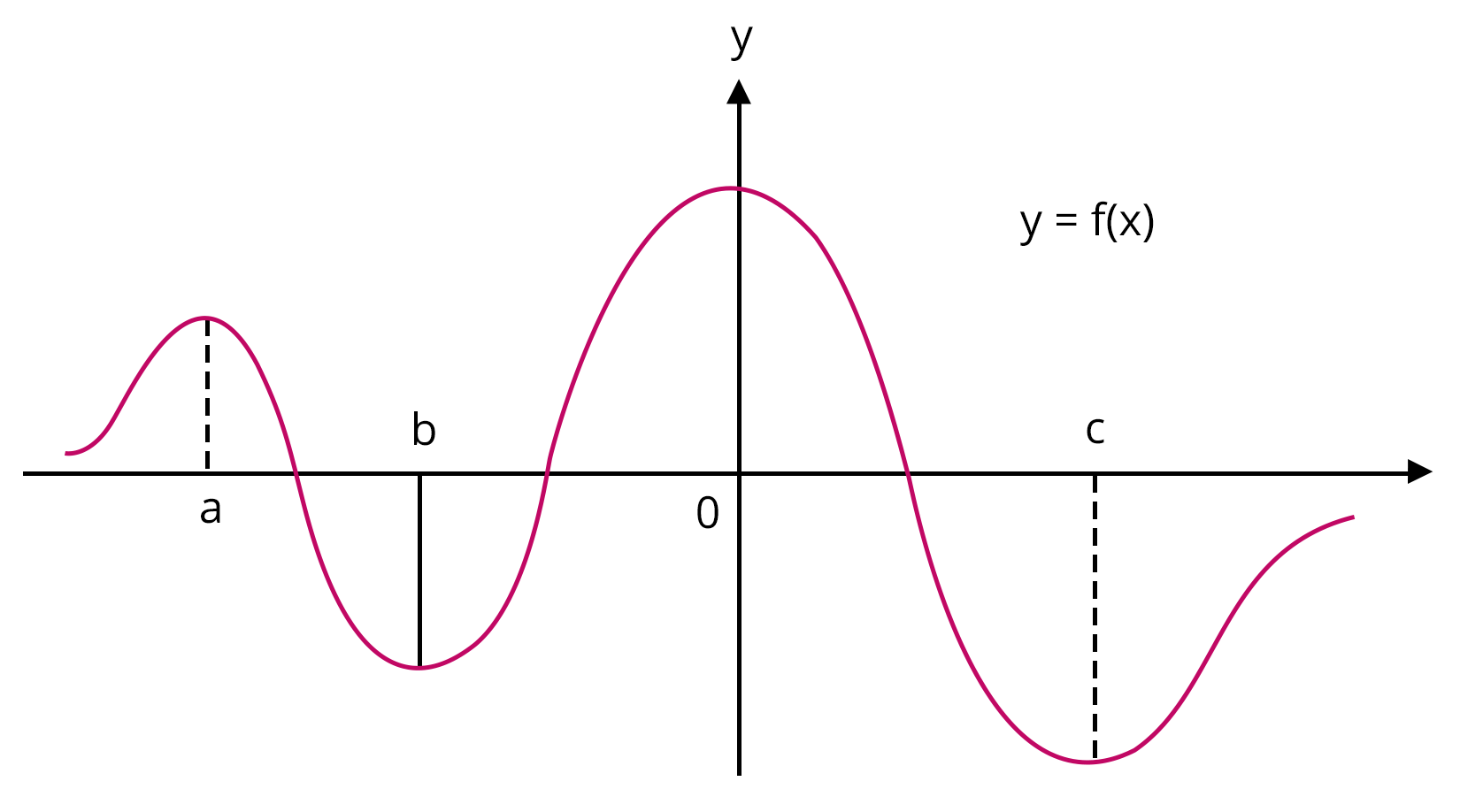Image: Maxima and Minima

4. Tangents and Normals

A tangent to a curve is a line that touches it at one point and has the same slope as the curve.

A line perpendicular to a tangent to the curve is called a normal to the curve.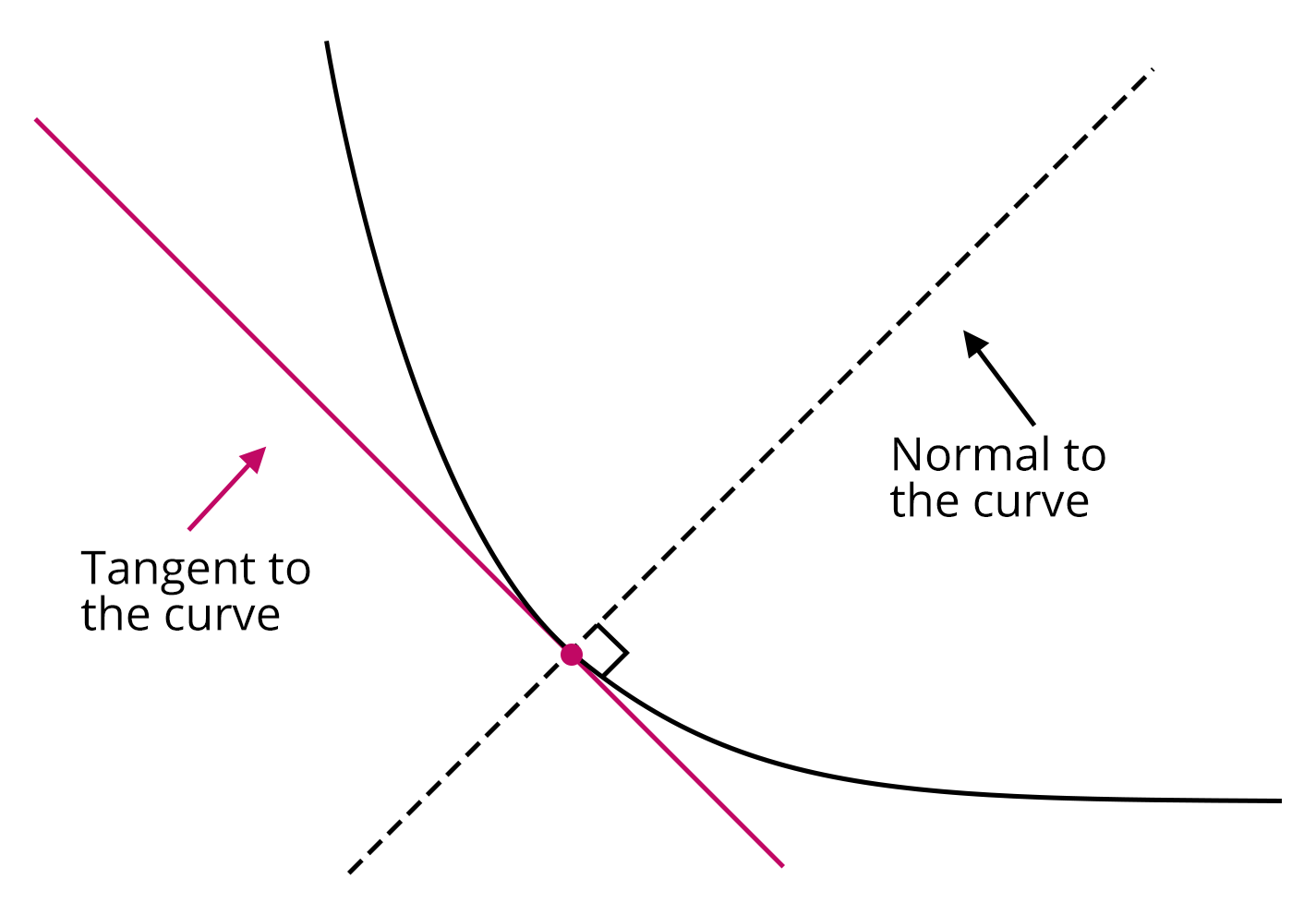Image: Tangent and Normal

### Examples on Limit and Continuity

Example on Limit:

Evaluate $\underset{x\to 0}{\lim} \dfrac{x\cos{(x)}-\sin{(x)}}{x^2\sin{(x)}}$

Solution: Here the given limit is of the form $\dfrac{0}{0}$

Using L’Hospital Rule and differentiating, we get

$\Rightarrow \underset{x \to 0}{\lim} \dfrac{\cos{(x)}-x\sin{(x)}-\cos{(x)}}{x^2\cos{(x)} + 2x\sin{(x)}}$

$\Rightarrow \underset{x \to 0}{\lim} \dfrac{-\sin{(x)}}{x\cos{(x)} + 2\sin{(x)}}$

$\Rightarrow \underset{x \to 0}{\lim} \dfrac{\dfrac{-\sin{(x)}}{x}}{\cos{(x)} + \dfrac{2\sin{(x)}}{x}}$

$\Rightarrow \dfrac{-1}{1 + 2(1)}$

$\Rightarrow \dfrac{-1}{3}$

Example on Continuity:

Find the continuity of the function f(x) = 3x + 4 at the point x = 5.

Solution: The given function is f(x) = 3x + 4, and its value at the point x = 5 is f(5) = 19.

Let us find the limit of the function at the point x = 5.

$\underset{x \to 5}{\lim}f(x)$

$\Rightarrow \underset{x \to 5}{\lim} (3x + 4)$

$\Rightarrow 3(5) + 4$

$\Rightarrow 15 + 4 = 19 = f(5)$

Therefore, the function f(x) is continuous at the point x = 5.

### Solved problems of Previous Year’s Question

1. If $f(1)=1, f^{\prime}(1)=2$ then $\underset{x \rightarrow 1}{\lim} \dfrac{\sqrt{f(x)}-1}{\sqrt{x}-1}$ is

Ans: Given function is $\underset{x \rightarrow 1}{\lim} \dfrac{\sqrt{f(x)}-1}{\sqrt{x}-1}$ and the limit is of the form (0/0)

Using L’Hospital's rule we get,

$\Rightarrow \underset{x \rightarrow 1}{\lim} \dfrac{\dfrac{1}{2 \sqrt{f(x)}} f^{\prime}(x)}{\dfrac{1}{2 \sqrt{x}}}$

$\Rightarrow \dfrac{f^{\prime}(1)}{\sqrt{f(1)}}$

As it is given that $f(1)=1, f^{\prime}(1)=2$

Hence $\dfrac{f^{\prime}(1)}{\sqrt{f(1)}}=2$

2. $f(x)$ and $g(x)$ are two differentiable functions on $\left[0,2\right]$ such that $f^{\prime \prime}(x)-g^{\prime \prime}(x)=0$, $f^{\prime \prime}(1)=2 g^{\prime}(1)=4 f(2)=3 g(2)=9$ then $f(x)-g(x)$ at $x=3 / 2$ is

Ans: Given $f^{\prime \prime}(x)-g^{\prime \prime}(x)=0$

On Integrating the above equation we get,